# Dijkstra Algorithm | Example | Time Complexity

## Dijkstra Algorithm-

• Dijkstra Algorithm is a very famous greedy algorithm.
• It is used for solving the single source shortest path problem.
• It computes the shortest path from one particular source node to all other remaining nodes of the graph.

## Conditions-

It is important to note the following points regarding Dijkstra Algorithm-

• Dijkstra algorithm works only for connected graphs.
• Dijkstra algorithm works only for those graphs that do not contain any negative weight edge.
• The actual Dijkstra algorithm does not output the shortest paths.
• It only provides the value or cost of the shortest paths.
• By making minor modifications in the actual algorithm, the shortest paths can be easily obtained.
• Dijkstra algorithm works for directed as well as undirected graphs.

## Dijkstra Algorithm-

```dist[S] ← 0                                                  // The distance to source vertex is set to 0

Π[S] ← NIL                                                   // The predecessor of source vertex is set as NIL

for all v ∈ V - {S}                                          // For all other vertices

do dist[v] ← ∞                                       // All other distances are set to ∞

Π[v] ← NIL                                           // The predecessor of all other vertices is set as NIL

S ← ∅                                                        // The set of vertices that have been visited 'S' is initially empty

Q ← V                                                        // The queue 'Q' initially contains all the vertices

while Q ≠ ∅                                                  // While loop executes till the queue is not empty

do u ← mindistance (Q, dist)                         // A vertex from Q with the least distance is selected

S ← S ∪ {u}                                          // Vertex 'u' is added to 'S' list of vertices that have been visited

for all v ∈ neighbors[u]                                     // For all the neighboring vertices of vertex 'u'

do if dist[v] > dist[u] + w(u,v)                     // if any new shortest path is discovered

then dist[v] ← dist[u] + w(u,v)              // The new value of the shortest path is selected

return dist```

## Implementation-

The implementation of above Dijkstra Algorithm is explained in the following steps-

### Step-01:

In the first step. two sets are defined-

• One set contains all those vertices which have been included in the shortest path tree.
• In the beginning, this set is empty.
• Other set contains all those vertices which are still left to be included in the shortest path tree.
• In the beginning, this set contains all the vertices of the given graph.

### Step-02:

For each vertex of the given graph, two variables are defined as-

• Π[v] which denotes the predecessor of vertex ‘v’
• d[v] which denotes the shortest path estimate of vertex ‘v’ from the source vertex.

Initially, the value of these variables is set as-

• The value of variable ‘Π’ for each vertex is set to NIL i.e. Π[v] = NIL
• The value of variable ‘d’ for source vertex is set to 0 i.e. d[S] = 0
• The value of variable ‘d’ for remaining vertices is set to ∞ i.e. d[v] = ∞

### Step-03:

The following procedure is repeated until all the vertices of the graph are processed-

• Among unprocessed  vertices, a vertex with minimum value of variable ‘d’ is chosen.
• Its outgoing edges are relaxed.
• After relaxing the edges for that vertex, the sets created in step-01 are updated.

### What is Edge Relaxation?

Consider the edge (a,b) in the following graph-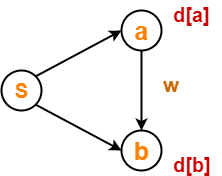Here, d[a] and d[b] denotes the shortest path estimate for vertices a and b respectively from the source vertex ‘S’.

Now,

If d[a] + w < d[b]

then d[b] = d[a] + w and Π[b] = a

This is called as edge relaxation.

## Time Complexity Analysis-

### Case-01:

This case is valid when-

• The given graph G is represented as an adjacency matrix.
• Priority queue Q is represented as an unordered list.

Here,

• A[i,j] stores the information about edge (i,j).
• Time taken for selecting i with the smallest dist is O(V).
• For each neighbor of i, time taken for updating dist[j] is O(1) and there will be maximum V neighbors.
• Time taken for each iteration of the loop is O(V) and one vertex is deleted from Q.
• Thus, total time complexity becomes O(V2).

### Case-02:

This case is valid when-

• The given graph G is represented as an adjacency list.
• Priority queue Q is represented as a binary heap.

Here,

• With adjacency list representation, all vertices of the graph can be traversed using BFS in O(V+E) time.
• In min heap, operations like extract-min and decrease-key value takes O(logV) time.
• So, overall time complexity becomes O(E+V) x O(logV) which is O((E + V) x logV) = O(ElogV)
• This time complexity can be reduced to O(E+VlogV) using Fibonacci heap.

## Problem-

Using Dijkstra’s Algorithm, find the shortest distance from source vertex ‘S’ to remaining vertices in the following graph-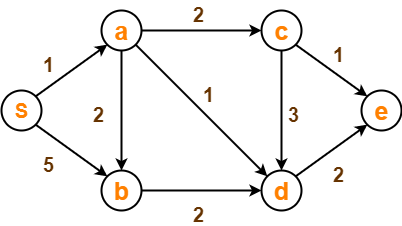Also, write the order in which the vertices are visited.

## Solution-

### Step-01:

The following two sets are created-

• Unvisited set : {S , a , b , c , d , e}
• Visited set     : { }

### Step-02:

The two variables  Π and d are created for each vertex and initialized as-

• Π[S] = Π[a] = Π[b] = Π[c] = Π[d] = Π[e] = NIL
• d[S] = 0
• d[a] = d[b] = d[c] = d[d] = d[e] = ∞

### Step-03:

• Vertex ‘S’ is chosen.
• This is because shortest path estimate for vertex ‘S’ is least.
• The outgoing edges of vertex ‘S’ are relaxed.

#### Before Edge Relaxation-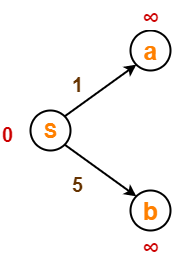Now,

• d[S] + 1 = 0 + 1 = 1 < ∞

∴ d[a] = 1 and Π[a] = S

• d[S] + 5 = 0 + 5 = 5 < ∞

∴ d[b] = 5 and Π[b] = S

After edge relaxation, our shortest path tree is-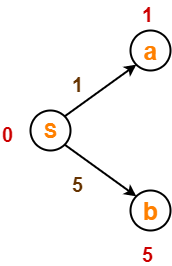Now, the sets are updated as-

• Unvisited set : {a , b , c , d , e}
• Visited set : {S}

### Step-04:

• Vertex ‘a’ is chosen.
• This is because shortest path estimate for vertex ‘a’ is least.
• The outgoing edges of vertex ‘a’ are relaxed.

#### Before Edge Relaxation-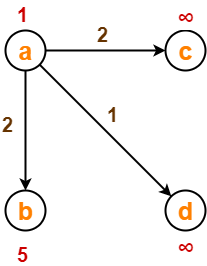Now,

• d[a] + 2 = 1 + 2 = 3 < ∞

∴ d[c] = 3 and Π[c] = a

• d[a] + 1 = 1 + 1 = 2 < ∞

∴ d[d] = 2 and Π[d] = a

• d[b] + 2 = 1 + 2 = 3 < 5

∴ d[b] = 3 and Π[b] = a

After edge relaxation, our shortest path tree is-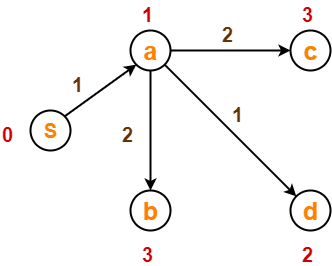Now, the sets are updated as-

• Unvisited set : {b , c , d , e}
• Visited set : {S , a}

### Step-05:

• Vertex ‘d’ is chosen.
• This is because shortest path estimate for vertex ‘d’ is least.
• The outgoing edges of vertex ‘d’ are relaxed.

#### Before Edge Relaxation-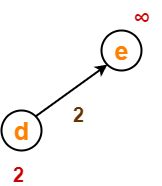Now,

• d[d] + 2 = 2 + 2 = 4 < ∞

∴ d[e] = 4 and Π[e] = d

After edge relaxation, our shortest path tree is-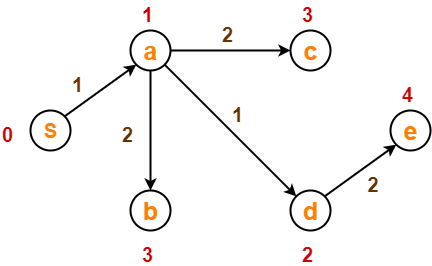Now, the sets are updated as-

• Unvisited set : {b , c , e}
• Visited set : {S , a , d}

### Step-06:

• Vertex ‘b’ is chosen.
• This is because shortest path estimate for vertex ‘b’ is least.
• Vertex ‘c’ may also be chosen since for both the vertices, shortest path estimate is least.
• The outgoing edges of vertex ‘b’ are relaxed.

#### Before Edge Relaxation-Now,

• d[b] + 2 = 3 + 2 = 5 > 2

∴ No change

After edge relaxation, our shortest path tree remains the same as in Step-05.

Now, the sets are updated as-

• Unvisited set : {c , e}
• Visited set     : {S , a , d , b}

### Step-07:

• Vertex ‘c’ is chosen.
• This is because shortest path estimate for vertex ‘c’ is least.
• The outgoing edges of vertex ‘c’ are relaxed.

#### Before Edge Relaxation-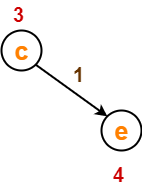Now,

• d[c] + 1 = 3 + 1 = 4 = 4

∴ No change

After edge relaxation, our shortest path tree remains the same as in Step-05.

Now, the sets are updated as-

• Unvisited set : {e}
• Visited set : {S , a , d , b , c}

### Step-08:

• Vertex ‘e’ is chosen.
• This is because shortest path estimate for vertex ‘e’ is least.
• The outgoing edges of vertex ‘e’ are relaxed.
• There are no outgoing edges for vertex ‘e’.
• So, our shortest path tree remains the same as in Step-05.

Now, the sets are updated as-

• Unvisited set : { }
• Visited set : {S , a , d , b , c , e}

Now,

• All vertices of the graph are processed.
• Our final shortest path tree is as shown below.
• It represents the shortest path from source vertex ‘S’ to all other remaining vertices.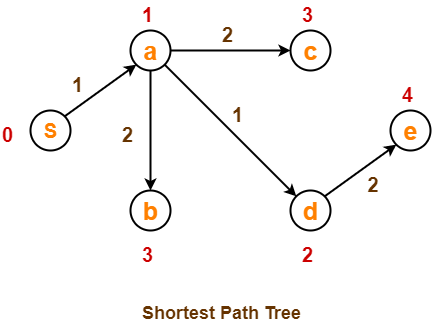The order in which all the vertices are processed is :

S , a , d , b , c , e.

To gain better understanding about Dijkstra Algorithm,

Watch this Video Lecture

Next Article- Floyd-Warshall Algorithm

Get more notes and other study material of Design and Analysis of Algorithms.

Watch video lectures by visiting our YouTube channel LearnVidFun.

SummaryArticle Name
Dijkstra Algorithm | Example | Time Complexity
Description
Dijkstra Algorithm is a Greedy algorithm for solving the single source shortest path problem. Dijkstra Algorithm Example, Pseudo Code, Time Complexity, Implementation & Problem.
Author
Publisher Name
Gate Vidyalay
Publisher Logo